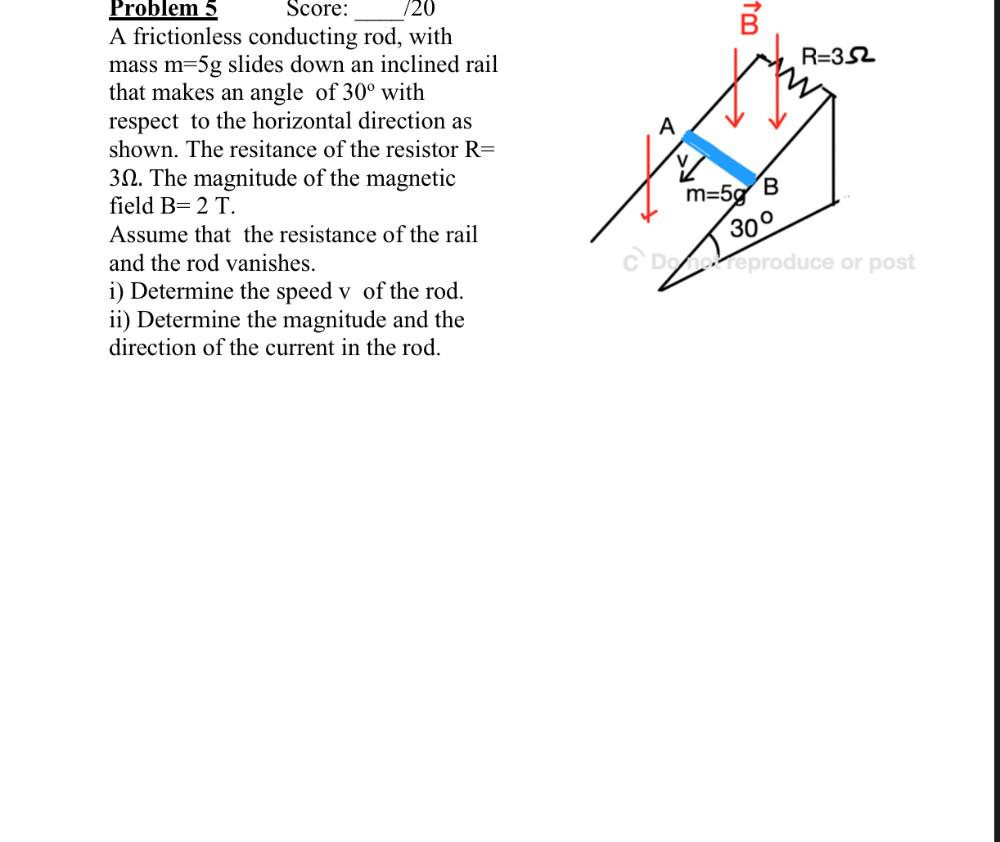Question:

# B R=352 Problem 5 Score: 720 A frictionless conducting rod, with mass m=5g slides down an inclined rail that makes an angle of 3B R=352 Problem 5 Score: 720 A frictionless conducting rod, with mass m=5g slides down an inclined rail that makes an angle of 30° with respect to the horizontal direction as shown. The resitance of the resistor R= 312. The magnitude of the magnetic field B= 2 T. Assume that the resistance of the rail and the rod vanishes. i) Determine the speed v of the rod. ii) Determine the magnitude and the direction of the current in the rod. m=5g B 300 CD2 Veproduce or post